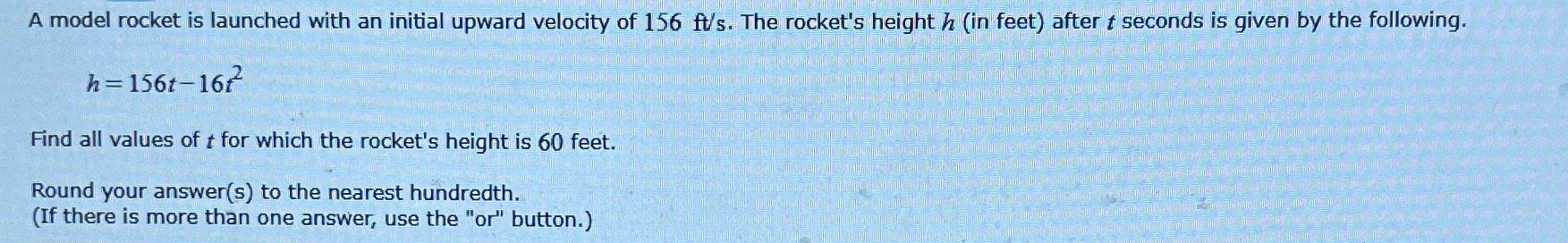### ¿Todavía tienes preguntas de matemáticas?

Pregunte a nuestros tutores expertos
Algebra
PreguntaA model rocket is launched with an initial upward velocity of $$156 ft / s$$ . The rocket's height $$h$$ (in feet) after $$t$$ seconds is given by the following. $$h = 156 t - 16 t ^ { 2 }$$ .Find all values of $$t$$ for which the rocket's height is $$60$$ feet. Round your answer(s) to the nearest hundredth. (If there is more than one answer, use the "or" button.)